In this section the distribution of time-codes across a SpaceWire network is described.

The initial state of a network is shown in Figure 66. N1 and N2 are nodes. R1 and R2 are routers. The dotted lines represent the SpaceWire links between the nodes and routers. N1 is time master and sends out a time-code whenever its TICK_IN signal is asserted. The numbers in the node and router boxes represent the current values of their time-counters.Figure 66 Initial State of Network

When TICK_IN is asserted in node N1, its time-counter is incremented from 40 to 41 and a time-code with time value 41 is transmitted. This is shown in Figure 67.Figure 67 TICK_IN Asserted

The transmitted time-code is received by router R1 which checks that the time value is one more than current time counter value, and then increments its time-counter and sends out time-code on all its other links. See Figure 68.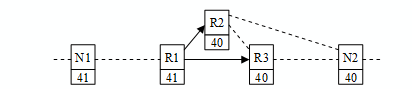Figure 68 Router R1 Forwards Time-Codes

Routers R2 and R3 both receive time-codes sent from R1 and both of these routers send out time-code on all other links as shown in Figure 69.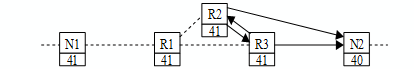Figure 69 Routers R2 and R3 Forward Time-Codes

Node N2 receives the time-code from R3 and validates that the incoming time-code has a time value of one more than the node’s time-counter. It then increments the time-counter and asserts TICK-OUT. See Figure 70.

Time in N2 is updated.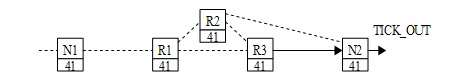Figure 70 N2 Receives Time-Code and Asserts TICK_OUT

R3 will also receive a time-code from R2, which is now not one more than R3’s time-counter value, so is ignored as shown in Figure 71. This prevents time-codes from going back through the network. Time-codes move forward through the network even when there are loops in the network.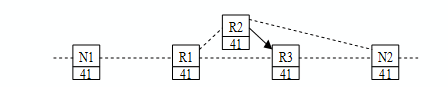Figure 71 Time-Codes Cannot Go Backwards

Node N2 will also receive a time-code from R2 which is not one more than N2’s time-counter value, so is ignored. This prevents multiple triggering of the TICK_OUT signal as duplicate copies of the time-code which have taken different paths through the SpaceWire network arrive at the node. See Figure 72.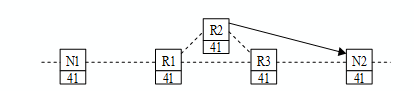Figure 72 Other Time-Codes Received at N2 are Not Valid